……

站在2019年的起点上，回望哈弗的2018年，这一年，立志成为“中国SUV全球领导者”的哈弗取得了有目共睹的丰硕成果，厚重的成绩单不仅为哈弗的2018画上了一个圆满的句号，其中500万销量的达成更成为了哈弗品牌发展史上的一个重要里程碑。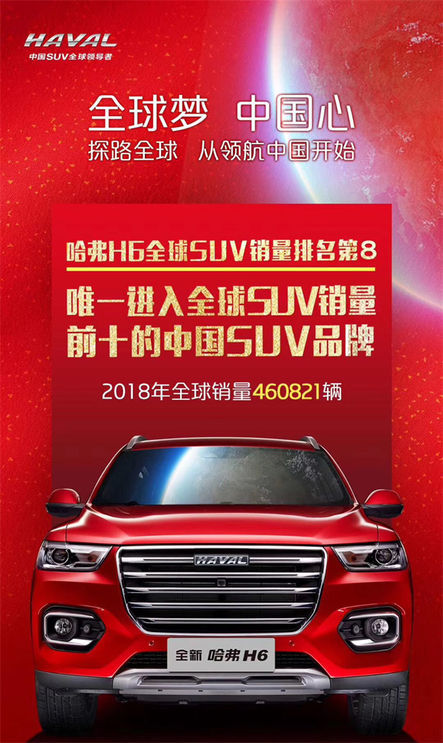收获与喜悦的背后，是哈弗13年来对造好车、造专业SUV初心的坚守，而要坚守这颗初心，靠的是哈弗在技术创新方面的真功夫、真实力。因为打造具有全球竞争力的产品，成为具有全球影响力的中国自主品牌，是所有哈弗人为之奋进的动力与目标。因此，在技术创新上，哈弗一直在路上，且成绩斐然。

坚持技术创新引领 打造坚不可摧的“品质堡垒” 成就哈弗SUV霸主地位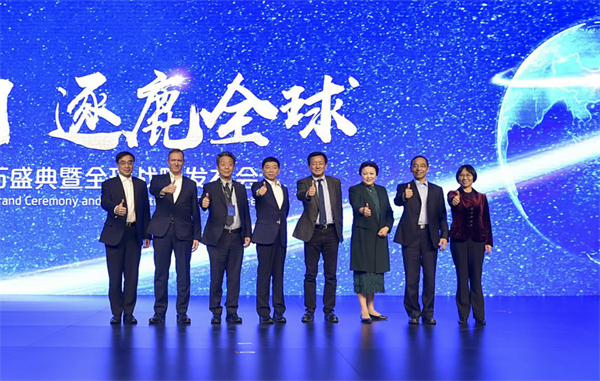当下，随着中国经济进入深度转型期，中国品牌全球化已经成为一个必然趋势。而在实现全球化战略的道路上，哈弗始终将技术创新作为品牌可持续发展的根本。正如长城汽车总裁王凤英在哈弗全球500万盛典上所说：“科技创新，我们一直都是在投资的路上，一直都是无止境的研发投入。”

哈弗是这么说的，也是这么做的。自品牌诞生以来，哈弗在技术研发上一直坚持“过度投入”战略。在该战略指引下，哈弗在发动机、变速器、整车造型、整车设计等关键核心技术方面均取得了突破性的成果。尤其在决定车辆品质最核心的动力总成技术上，哈弗的“双十佳”动力总成技术已经达到了国际一流水准，占据了绝对领先优势，树立了中国自主品牌技术创新的典范。其中1.5GDIT 发动机一举斩获“中国心”年度十佳发动机称号，拥有91项专利，特别是该发动机采用的CVVL连续可变气门升程技术在全球车企中首屈一指，目前也只有宝马、英菲尼迪等国际汽车品牌具有类似的发动机技术。与之相匹配的7DCT湿式双离合变速器更是彻底填补了中国品牌在7速湿式双离合变速器研发方面的空白，是中国自主品牌在技术革新上的一次质的飞跃。该变速器荣获了“世界十佳变速器”的殊荣。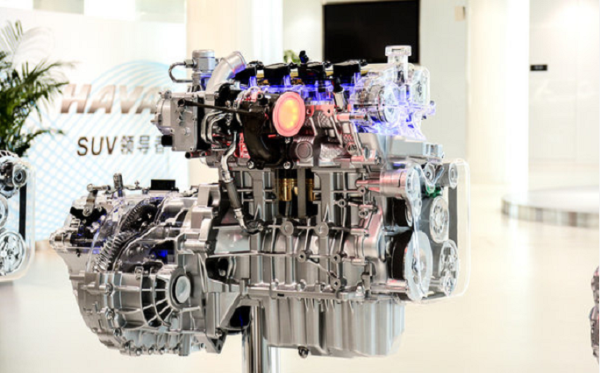不止如此，在安全方面，哈弗在不断创新中摸索出了一套独特的全方位智能化安全体系，建立了同类SUV产品中安全性能方面的绝对领先优势。经过多年的口碑累积，哈弗已经在广大消费者心目中树立了“买哈弗，就是买最安全SUV”的良好品牌形象。

13年来，正是凭借着对技术创新引领始终如一的坚持，哈弗打造出了一座坚不可摧的“品质堡垒” ，全速领跑中国SUV市场，并赢得了国际市场的高度认可。即使在2018年全球汽车市场整体增长放缓的大环境下，哈弗依然保持着稳健的增长势头：哈弗全球累计销量突破500万辆，成为中国首个进入500万俱乐部的专业SUV品牌，并一举创下了连续9年蝉联中国SUV销量第一的记录；“国民神车”哈弗H6则连续三年进入全球SUV销量榜前十，累计67个月成为SUV销量冠军。尽显哈弗SUV霸主本色。

与时俱进抢占“智”高点 哈弗开启智能互联新时代 夯实国际竞争实力

在朝着“中国SUV全球领导者”的目标奋进的道路上，哈弗坚持自主创新的同时，不忘与时俱进，并凭借着对市场和消费需求的精准洞察，抢先布局新技术领域。因为哈弗深知，唯有这样，才能在激烈的竞争中始终保持领先优势。

因此，当敏锐洞察到智能化将成为未来汽车消费的主流趋势时，哈弗抢先发力，积极布局智能化。仅在研发布局方面，哈弗就在全球建立了包括智能驾驶、智能网联等八个研发中心，汇集了全球400 多位顶尖汽车工程师、设计师和IT专家，组建了最豪华、最具全球化的自主研发团队。这为哈弗打造最具全球竞争力的智能化产品提供了领先的技术支持。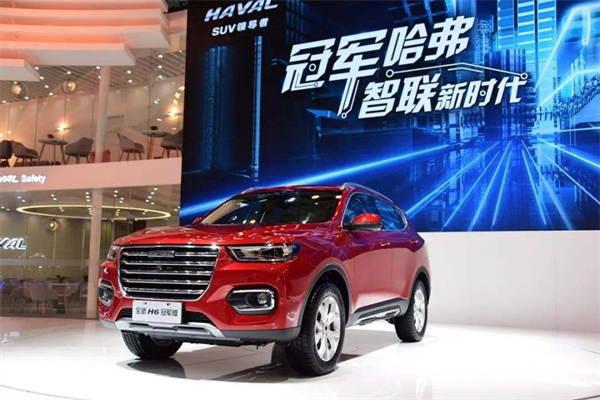哈弗也因此在智能化领域处于行业领先地位。尤其在智能网联研发上，哈弗以基础技术、用户思维、个性化服务，三位一体构建全场景化智能服务体系，配合万物互联平台，构建了一个5G全球智联生态，全面满足了全球24大语言区的用户需求，为用户提供了行业领先的、更智能化的产品体验。2018年上市的全新哈弗 H6 冠军版、哈弗 H4 智联版以及两款主打智能网联的F系产品，就是哈弗在智能化方面取得的最佳成果。H&F双系合璧，全面开启了哈弗智能新时代，引领了智能化消费新趋势。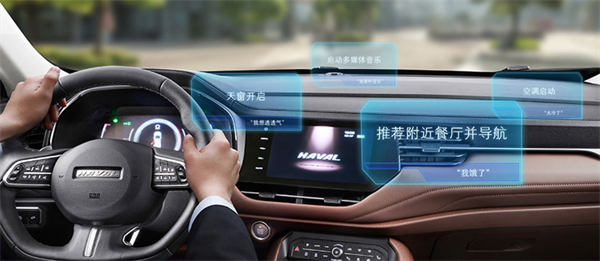正是这种对技术发展趋势的正确前瞻预判以及快速响应，进一步夯实了哈弗的霸主地位，大力提升了哈弗的国际竞争力，使得哈弗朝着“中国SUV全球领导者”的目标迈进的步伐更加坚定。

进击全球 哈弗创新不止 共享创新全球领先技术

在哈弗看来，技术创新永无止境，尤其面对复杂多变的市场环境，更应时刻把关注的焦点放在未来。因为成功总是留给有准备的人。因此，在代表哈弗2018年至高荣耀的哈弗全球500万盛典上，长城汽车董事长魏建军发布了哈弗“5-2-1”全球化战略，即哈弗利用5年时间，实现年度销200万台，成就哈弗全球专业SUV第一品牌。为此，哈弗在未来5年内的全球研发投入将超过300亿，并将在新能源、智能驾驶、智能网联、氢动力等未来主流发展领域持续深耕技术研发，与优秀的自主品牌共享创新全球技术。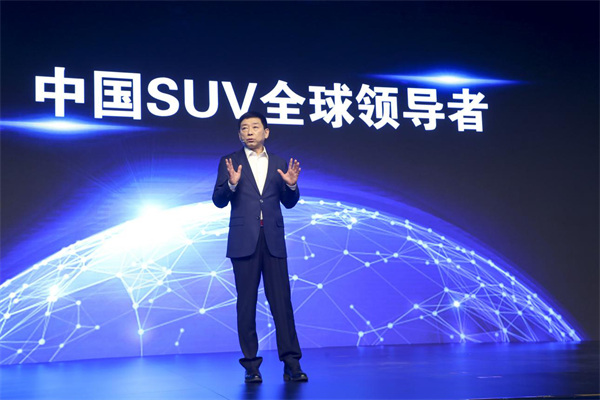其中，在新能源研发上，哈弗已形成领先优势，利用开发的第二代串联、并联混动技术，全力打造全球高品质的SUV产品。在FCEV领域的技术创新，更使哈弗氢动力研发处于全球领先。更值得一提的是，2022年哈弗氢动力车将在北京冬奥会正式亮相，向世界彰显中国自主创新实力。预计在2023年以前，哈弗还将推出20款具备新四化领先科技的全球产品。

值得一提的是，在盛典中魏建军还代表哈弗发起了中国车企全球联盟的倡议，愿与优秀的自主品牌，在新能源、智能驾驶、智能网联等所有领域进行深度合作，共享创新全球领先技术。

毫无疑问，哈弗的这一系列技术领先优势及开放共赢的倡议，将加速推进哈弗品牌乃至整个中国汽车全球化的进程，让中国从一个汽车引入大国变成一个汽车输出大国。

总结：

不谋万世者，不足谋一时；不谋全局者，不足谋一域。事实证明，哈弗成功做到了。13年来，在自主创新引领下，哈弗以定义者的身份开辟了SUV市场，以创变者的姿态持续引领着SUV市场。2018年，哈弗更是已拥有500万销量的绝对实力。这是全球消费者对哈弗的信赖，更是哈弗不断提高自主创新能力，实施技术革新的伟大成果。

2019年是哈弗全球化战略的出发元年。随着全球化战略的不断推进，哈弗立志要让中国制造成为全球高品质、高科技产品的代名词，开创新纪元，续写辉煌新篇章。

领跑中国，逐鹿全球，哈弗一直在路上！

##### 相关资讯

|分享

•已阅！握爪
•我手滑为你点赞
•128个赞！
•不明觉厉
•阅后既醉
•有钱！任性
•照片太美，我不敢看
•干货！杠杠的
•高大上
•膜拜中！
•请接受我的膝盖
•猫哥/猫妹么么哒
•神吐槽
•我只笑笑不说话
•我想静静
•窒息
##### 资讯相关车系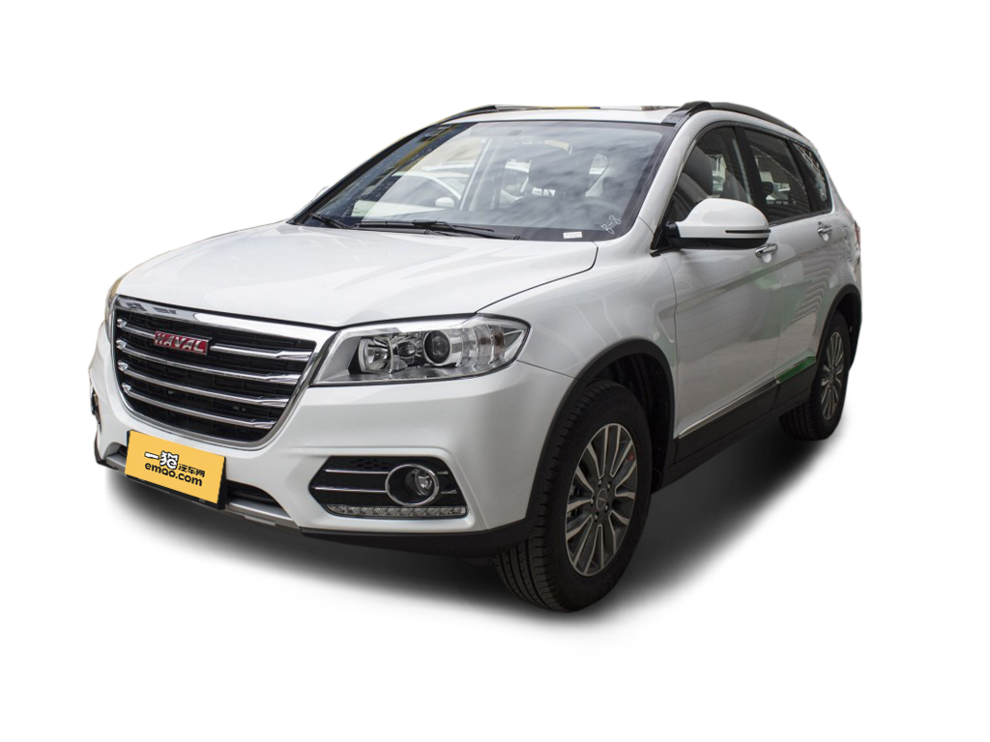##### 热度排行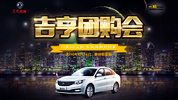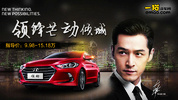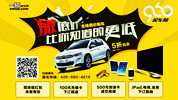• 阿斯顿·马丁
• 奥迪
• 阿尔法罗密欧
• ALPINA
• 爱驰
• AC Schnitzer

• 巴博斯
• 宝骏
• 宝马
• 保时捷
• 北汽制造
• 奔驰
• 奔腾
• 本田
• 比亚迪
• 标致
• 别克
• 宾利
• 布加迪
• 北汽威旺
• 北京
• 北汽绅宝
• 北汽幻速
• 北汽新能源
• 宝沃
• 比速汽车
• 北汽道达
• 北京汽车
• 比德文汽车
• 铂驰
• 北汽昌河

• 昌河
• 长安
• 长城
• 长安商用
• 成功汽车
• 长江EV
• 长安轻车型
• 长安欧尚
• 长安凯程
• 长安跨越
• 车驰汽车

• 大众
• 道奇
• 东风
• 东风风神
• 东风小康
• 东南
• DS
• 东风风行
• 东风风度
• 东风风光
• 电咖
• 大乘汽车
• 大运
• 东风富康

• 法拉利
• 菲亚特
• 丰田
• 福迪
• 福特
• 福田汽车
• 福汽启腾
• 风诺
• 福田

• GMC
• 广汽传祺
• 广汽吉奥
• 观致
• 国金汽车
• 广汽新能源
• 广汽集团
• 国机智骏

• 哈飞
• 海格
• 海马
• 华泰
• 黄海
• 恒天
• 红旗
• 哈弗
• 华颂
• 华凯
• 华泰新能源
• 汉腾汽车
• 华利
• 红星汽车
• HYCAN合创
• 汉龙汽车

• Jeep
• 江淮
• 江铃
• 捷豹
• 金杯
• 九龙
• 吉利汽车
• 金旅
• 金龙
• 江铃集团轻汽
• 江铃集团新能源
• 君马汽车
• 捷途
• 钧天
• 捷达
• 几何汽车

• 开瑞
• 凯迪拉克
• 科尼赛克
• 克莱斯勒
• KTM
• 卡威
• 凯翼
• 康迪
• 康迪电动汽车集团
• 开沃汽车
• 卡升

• 猎豹汽车
• 兰博基尼
• 劳斯莱斯
• 雷克萨斯
• 雷诺
• 理念
• 力帆
• 莲花汽车
• 林肯
• 铃木
• 陆风
• 路虎
• 路特斯
• 领志
• 领克
• 零跑汽车
• Lorinser
• LOCAL MOTORS
• LITE
• 领途汽车
• 理想汽车
• 罗夫哈特
• 力帆汽车

• MG
• MINI
• 马自达
• 玛莎拉蒂
• 迈凯伦
• 摩根
• 名爵
• 迈莎锐
• 迈迈

• 纳智捷
• 哪吒汽车

• 讴歌
• 欧宝
• 欧朗
• 欧拉
• 欧尚汽车

• 帕加尼
• Polestar极星

• 奇瑞
• 启辰
• 起亚
• 前途
• 庆铃汽车
• 骐铃汽车
• 全球鹰
• 乔治·巴顿

• 日产
• 荣威
• 瑞麒汽车
• 如虎
• 瑞驰
• 瑞麒
• 瑞驰新能源
• 容大智造

• 上汽大通
• smart
• 三菱
• 双环
• 双龙
• 斯巴鲁
• 斯柯达
• 萨博
• 思铭
• 赛麟
• SWM斯威汽车
• 上汽MAXUS
• SRM鑫源
• 思皓
• SHELBY
• 上喆

• TESLA
• 泰卡特
• 腾势
• 特斯拉
• 天际汽车

• 威麟
• 威兹曼
• 沃尔沃
• 五菱汽车
• 五十铃
• 潍柴英致
• WEY
• 蔚来
• 威马汽车
• 潍柴汽车

• 现代
• 雪佛兰
• 雪铁龙
• 西雅特
• 新特汽车
• 小鹏汽车
• 新宝骏

• 野马汽车
• 一汽
• 依维柯
• 英菲尼迪
• 永源
• 驭胜
• 云度
• 宇通客车
• 云雀汽车
• 远程汽车
• 一汽凌河

• 中华
• 中兴
• 众泰
• 知豆
• 之诺
• 正道汽车
• A
• B
• C
• D
• E
• F
• G
• H
• I
• J
• K
• L
• M
• N
• O
• P
• Q
• R
• S
• T
• U
• V
• W
• X
• Y
• Z

• 阿斯顿·马丁
• 奥迪
• 阿尔法罗密欧
• ALPINA
• 爱驰
• AC Schnitzer

• 巴博斯
• 宝骏
• 宝马
• 保时捷
• 北汽制造
• 奔驰
• 奔腾
• 本田
• 比亚迪
• 标致
• 别克
• 宾利
• 布加迪
• 北汽威旺
• 北京
• 北汽绅宝
• 北汽幻速
• 北汽新能源
• 宝沃
• 比速汽车
• 北汽道达
• 北京汽车
• 比德文汽车
• 铂驰
• 北汽昌河

• 昌河
• 长安
• 长城
• 长安商用
• 成功汽车
• 长江EV
• 长安轻车型
• 长安欧尚
• 长安凯程
• 长安跨越
• 车驰汽车

• 大众
• 道奇
• 东风
• 东风风神
• 东风小康
• 东南
• DS
• 东风风行
• 东风风度
• 东风风光
• 电咖
• 大乘汽车
• 大运
• 东风富康

• 法拉利
• 菲亚特
• 丰田
• 福迪
• 福特
• 福田汽车
• 福汽启腾
• 风诺
• 福田

• GMC
• 广汽传祺
• 广汽吉奥
• 观致
• 国金汽车
• 广汽新能源
• 广汽集团
• 国机智骏

• 哈飞
• 海格
• 海马
• 华泰
• 黄海
• 恒天
• 红旗
• 哈弗
• 华颂
• 华凯
• 华泰新能源
• 汉腾汽车
• 华利
• 红星汽车
• HYCAN合创
• 汉龙汽车

• Jeep
• 江淮
• 江铃
• 捷豹
• 金杯
• 九龙
• 吉利汽车
• 金旅
• 金龙
• 江铃集团轻汽
• 江铃集团新能源
• 君马汽车
• 捷途
• 钧天
• 捷达
• 几何汽车

• 开瑞
• 凯迪拉克
• 科尼赛克
• 克莱斯勒
• KTM
• 卡威
• 凯翼
• 康迪
• 康迪电动汽车集团
• 开沃汽车
• 卡升

• 猎豹汽车
• 兰博基尼
• 劳斯莱斯
• 雷克萨斯
• 雷诺
• 理念
• 力帆
• 莲花汽车
• 林肯
• 铃木
• 陆风
• 路虎
• 路特斯
• 领志
• 领克
• 零跑汽车
• Lorinser
• LOCAL MOTORS
• LITE
• 领途汽车
• 理想汽车
• 罗夫哈特
• 力帆汽车

• MG
• MINI
• 马自达
• 玛莎拉蒂
• 迈凯伦
• 摩根
• 名爵
• 迈莎锐
• 迈迈

• 纳智捷
• 哪吒汽车

• 讴歌
• 欧宝
• 欧朗
• 欧拉
• 欧尚汽车

• 帕加尼
• Polestar极星

• 奇瑞
• 启辰
• 起亚
• 前途
• 庆铃汽车
• 骐铃汽车
• 全球鹰
• 乔治·巴顿

• 日产
• 荣威
• 瑞麒汽车
• 如虎
• 瑞驰
• 瑞麒
• 瑞驰新能源
• 容大智造

• 上汽大通
• smart
• 三菱
• 双环
• 双龙
• 斯巴鲁
• 斯柯达
• 萨博
• 思铭
• 赛麟
• SWM斯威汽车
• 上汽MAXUS
• SRM鑫源
• 思皓
• SHELBY
• 上喆

• TESLA
• 泰卡特
• 腾势
• 特斯拉
• 天际汽车

• 威麟
• 威兹曼
• 沃尔沃
• 五菱汽车
• 五十铃
• 潍柴英致
• WEY
• 蔚来
• 威马汽车
• 潍柴汽车

• 现代
• 雪佛兰
• 雪铁龙
• 西雅特
• 新特汽车
• 小鹏汽车
• 新宝骏

• 野马汽车
• 一汽
• 依维柯
• 英菲尼迪
• 永源
• 驭胜
• 云度
• 宇通客车
• 云雀汽车
• 远程汽车
• 一汽凌河

• 中华
• 中兴
• 众泰
• 知豆
• 之诺
• 正道汽车
• A
• B
• C
• D
• E
• F
• G
• H
• I
• J
• K
• L
• M
• N
• O
• P
• Q
• R
• S
• T
• U
• V
• W
• X
• Y
• Z

• 阿斯顿·马丁
• 奥迪
• 阿尔法罗密欧
• ALPINA
• 爱驰
• AC Schnitzer

• 巴博斯
• 宝骏
• 宝马
• 保时捷
• 北汽制造
• 奔驰
• 奔腾
• 本田
• 比亚迪
• 标致
• 别克
• 宾利
• 布加迪
• 北汽威旺
• 北京
• 北汽绅宝
• 北汽幻速
• 北汽新能源
• 宝沃
• 比速汽车
• 北汽道达
• 北京汽车
• 比德文汽车
• 铂驰
• 北汽昌河

• 昌河
• 长安
• 长城
• 长安商用
• 成功汽车
• 长江EV
• 长安轻车型
• 长安欧尚
• 长安凯程
• 长安跨越
• 车驰汽车

• 大众
• 道奇
• 东风
• 东风风神
• 东风小康
• 东南
• DS
• 东风风行
• 东风风度
• 东风风光
• 电咖
• 大乘汽车
• 大运
• 东风富康

• 法拉利
• 菲亚特
• 丰田
• 福迪
• 福特
• 福田汽车
• 福汽启腾
• 风诺
• 福田

• GMC
• 广汽传祺
• 广汽吉奥
• 观致
• 国金汽车
• 广汽新能源
• 广汽集团
• 国机智骏

• 哈飞
• 海格
• 海马
• 华泰
• 黄海
• 恒天
• 红旗
• 哈弗
• 华颂
• 华凯
• 华泰新能源
• 汉腾汽车
• 华利
• 红星汽车
• HYCAN合创
• 汉龙汽车

• Jeep
• 江淮
• 江铃
• 捷豹
• 金杯
• 九龙
• 吉利汽车
• 金旅
• 金龙
• 江铃集团轻汽
• 江铃集团新能源
• 君马汽车
• 捷途
• 钧天
• 捷达
• 几何汽车

• 开瑞
• 凯迪拉克
• 科尼赛克
• 克莱斯勒
• KTM
• 卡威
• 凯翼
• 康迪
• 康迪电动汽车集团
• 开沃汽车
• 卡升

• 猎豹汽车
• 兰博基尼
• 劳斯莱斯
• 雷克萨斯
• 雷诺
• 理念
• 力帆
• 莲花汽车
• 林肯
• 铃木
• 陆风
• 路虎
• 路特斯
• 领志
• 领克
• 零跑汽车
• Lorinser
• LOCAL MOTORS
• LITE
• 领途汽车
• 理想汽车
• 罗夫哈特
• 力帆汽车

• MG
• MINI
• 马自达
• 玛莎拉蒂
• 迈凯伦
• 摩根
• 名爵
• 迈莎锐
• 迈迈

• 纳智捷
• 哪吒汽车

• 讴歌
• 欧宝
• 欧朗
• 欧拉
• 欧尚汽车

• 帕加尼
• Polestar极星

• 奇瑞
• 启辰
• 起亚
• 前途
• 庆铃汽车
• 骐铃汽车
• 全球鹰
• 乔治·巴顿

• 日产
• 荣威
• 瑞麒汽车
• 如虎
• 瑞驰
• 瑞麒
• 瑞驰新能源
• 容大智造

• 上汽大通
• smart
• 三菱
• 双环
• 双龙
• 斯巴鲁
• 斯柯达
• 萨博
• 思铭
• 赛麟
• SWM斯威汽车
• 上汽MAXUS
• SRM鑫源
• 思皓
• SHELBY
• 上喆

• TESLA
• 泰卡特
• 腾势
• 特斯拉
• 天际汽车

• 威麟
• 威兹曼
• 沃尔沃
• 五菱汽车
• 五十铃
• 潍柴英致
• WEY
• 蔚来
• 威马汽车
• 潍柴汽车

• 现代
• 雪佛兰
• 雪铁龙
• 西雅特
• 新特汽车
• 小鹏汽车
• 新宝骏

• 野马汽车
• 一汽
• 依维柯
• 英菲尼迪
• 永源
• 驭胜
• 云度
• 宇通客车
• 云雀汽车
• 远程汽车
• 一汽凌河

• 中华
• 中兴
• 众泰
• 知豆
• 之诺
• 正道汽车
• A
• B
• C
• D
• E
• F
• G
• H
• I
• J
• K
• L
• M
• N
• O
• P
• Q
• R
• S
• T
• U
• V
• W
• X
• Y
• Z